# Get Started

Starting with rasterpic is very easy! You just need a image (png, jpeg/jpg or tif/tiff) and a spatial object (from the sf or the terra) package to start using it.

## Basic usage

We use here as an example the shape of Austria:

library(sf)
library(terra)
library(rasterpic)

# Plot
library(tidyterra)
library(ggplot2)

# Shape and image
x <- read_sf(system.file("gpkg/austria.gpkg", package = "rasterpic"))
img <- system.file("img/vertical.png", package = "rasterpic")

# Create!

default <- rasterpic_img(x, img)

autoplot(default) +
geom_sf(data = x)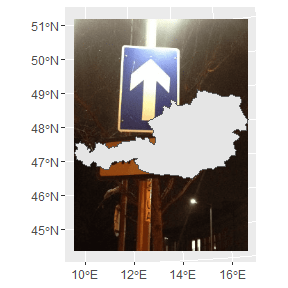## Options

The function provides several options for expanding, alignment and cropping.

### Expand

With this option the image is zoomed out of the spatial object:

expand <- rasterpic_img(x, img, expand = 1)

autoplot(expand) +
geom_sf(data = x)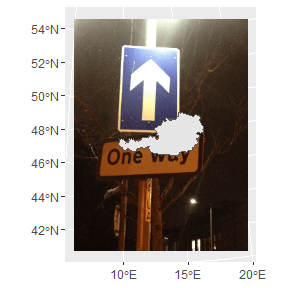### Alignment

Decide where to align the image:

bottom <- rasterpic_img(x, img, valign = 0)

autoplot(bottom) +
geom_sf(data = x)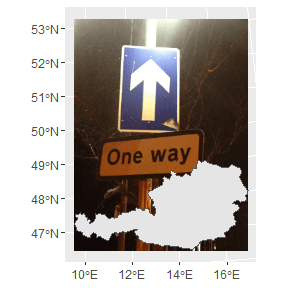Create impressive maps!:

mask <- rasterpic_img(x, img, crop = TRUE, mask = TRUE)

autoplot(mask)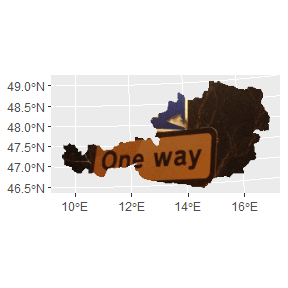maskinverse <- rasterpic_img(x, img, crop = TRUE, mask = TRUE, inverse = TRUE)

autoplot(maskinverse)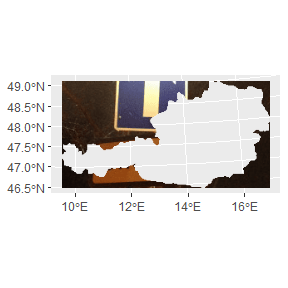• Spatial object of the sf package: sf, sfc, sfg or bbox.

• Spatial objects of the terra package: SpatRaster, SpatVector, SpatExtent.

• A vector of coordinates with the form c(xmin, ymin, xmax, yman)

rasterpic can parse the following image formats:
• png files.
• jpg/jpeg files.
• tif/tiff files.